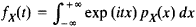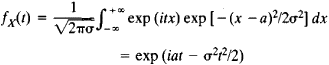# characteristic function

(redirected from Characteristic mapping)
Also found in: Dictionary.

## characteristic function

[‚kar·ik·tə′ris·tik ′fəŋk·shən]
(mathematics)
The function χA defined for any subset A of a set by setting χA (x) = 1 if x is in A and χA = 0 if x is not in A. Also known as indicator function.
(physics)
A function, such as the point characteristic function or the principal function, which is the integral of some property of an optical or mechanical system over time or over the path followed by the system, and whose value for a path actually followed by a system is a maximum or a minimum with respect to nearby paths with the same end points.
(statistics)
A function that uniquely defines a probability distribution; it is equal to √(2π) times the Fourier transform of the frequency function of the distribution.
McGraw-Hill Dictionary of Scientific & Technical Terms, 6E, Copyright © 2003 by The McGraw-Hill Companies, Inc.
The following article is from The Great Soviet Encyclopedia (1979). It might be outdated or ideologically biased.

## Characteristic Function

in mathematics:

(1) An eigenfunction.

(2) The characteristic, or indicator, function of a set A is a function f(x) that is defined on some set E containing A and that assumes the value f(x) = 1 if x is in A and the value f(x) = 0 if x is not in A.

(3) In probability theory, the characteristic function fx(t) of a random variable X is the mathematical expectation of the quantity exp (it X). For a random variable with probability density function PX(x), this definition yields the formulaFor example, for a random variable having a normal distribution with parameters a and σ, the characteristic function isThe characteristic function has several noteworthy properties. To every random variable X there corresponds a definite characteristic function. The probability distribution for X is uniquely determined by fX(t). When independent random variables are added, the corresponding characteristic functions are multiplied. If the concept of closeness is suitably defined, to random variables with close distributions there correspond characteristic functions that differ little from each other, and, conversely, to close characteristic functions there correspond random variables with close distributions. These properties underlie the applications of characteristic functions, particularly applications to the derivation of the limit theorems of probability theory.

A mathematical apparatus more or less equivalent to that of the characteristic function was first used by P. Laplace in 1812, but the full power of the characteristic function method was demonstrated in 1911 by A. M. Liapunov, who used the method in obtaining the theorem that bears his name.

The concept of characteristic function can be generalized to finite and infinite systems of random variables—that is, to random vectors and stochastic processes. The theory of characteristic functions has much in common with the theory of Fourier integrals.

### REFERENCES

Gnedenko, B. V. Kursteorii veroiatnostei, 5th ed. Moscow, 1969.
Prokhorov, Iu. V., and Iu. A. Rozanov. Teoriia veroiatnostei, 2nd ed. Moscow, 1973.